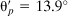## Answers to Selected Problems

### Chapter 1 Analysis of Stress

1.1. P = 4.27 kN

1.4. P = 32.5 kN, θ = 26.56°

1.5. σx′ = −13.15 MPa, τx′ y′ = −15.67 MPa

1.6. F = 67.32 kN/m3

1.9. Fx = Fy = Fz = 0

1.10. (a) σ1 = 121 MPa, σ = −71 MPa, τmax = 96 MPa

σ1 = 200 MPa, σ2 = −50 MPa, τmax = 125 MPa

(b)1.15. (a)σx = 38.68 MPa, σy = 12.12 MPa, τxy = 7MPa

(b)σ1 = 40.41 MPa, σ2 = 10.39 MPa,1.18. P = 3πpr2

1.24. (a)σ = −0.237τo, τ = 0.347τo

(b)σ1 = 3.732τo2 = −0.268τo,

1.26. σ1 = −σ2 = 51.96 MPa,

1.28. σ1 = 46.17 MPa, σ2 = 13.83 MPa,

1.30. (a) ...

Get Advanced Strength and Applied Elasticity, Fourth Edition now with the O’Reilly learning platform.

O’Reilly members experience live online training, plus books, videos, and digital content from nearly 200 publishers.